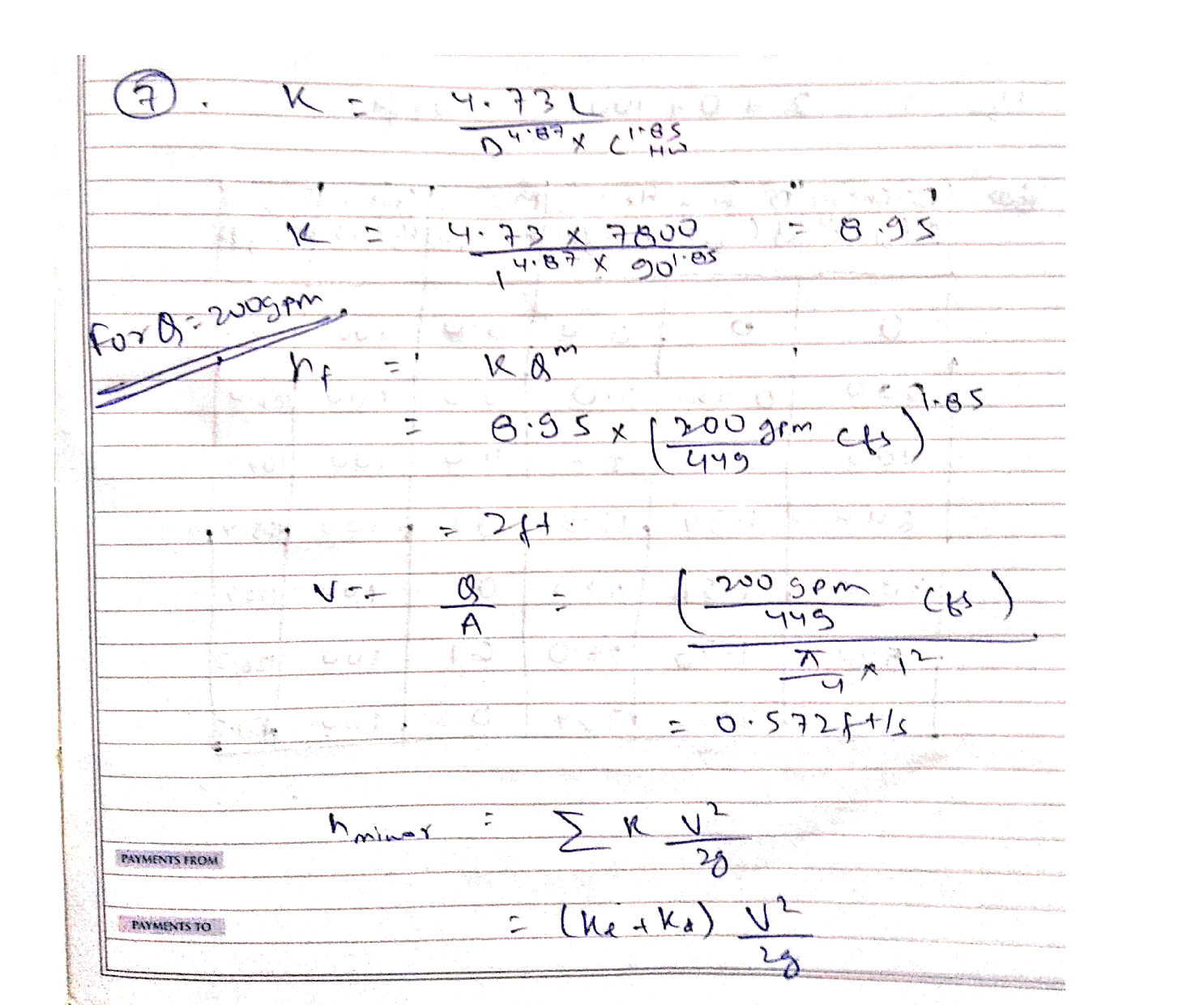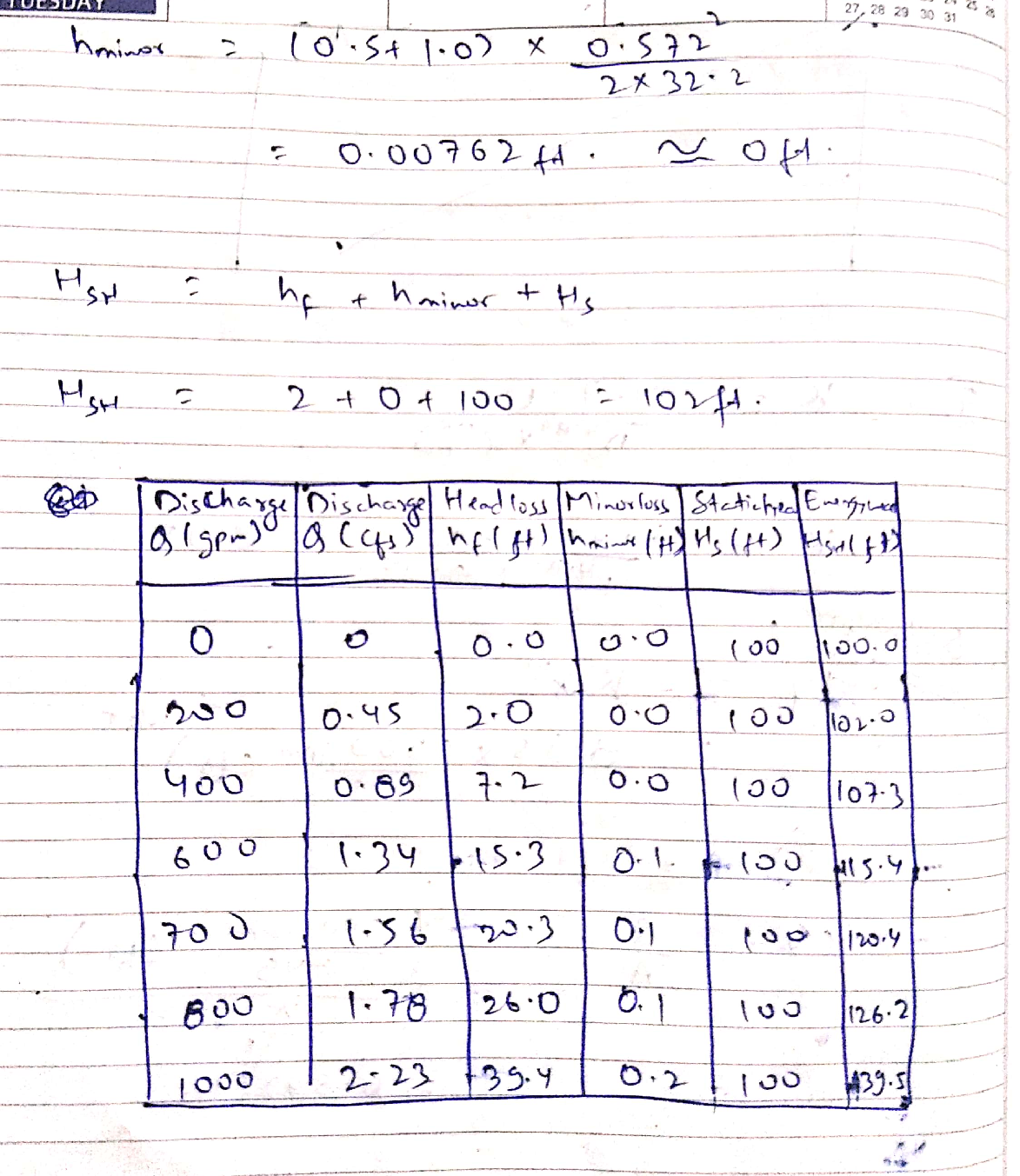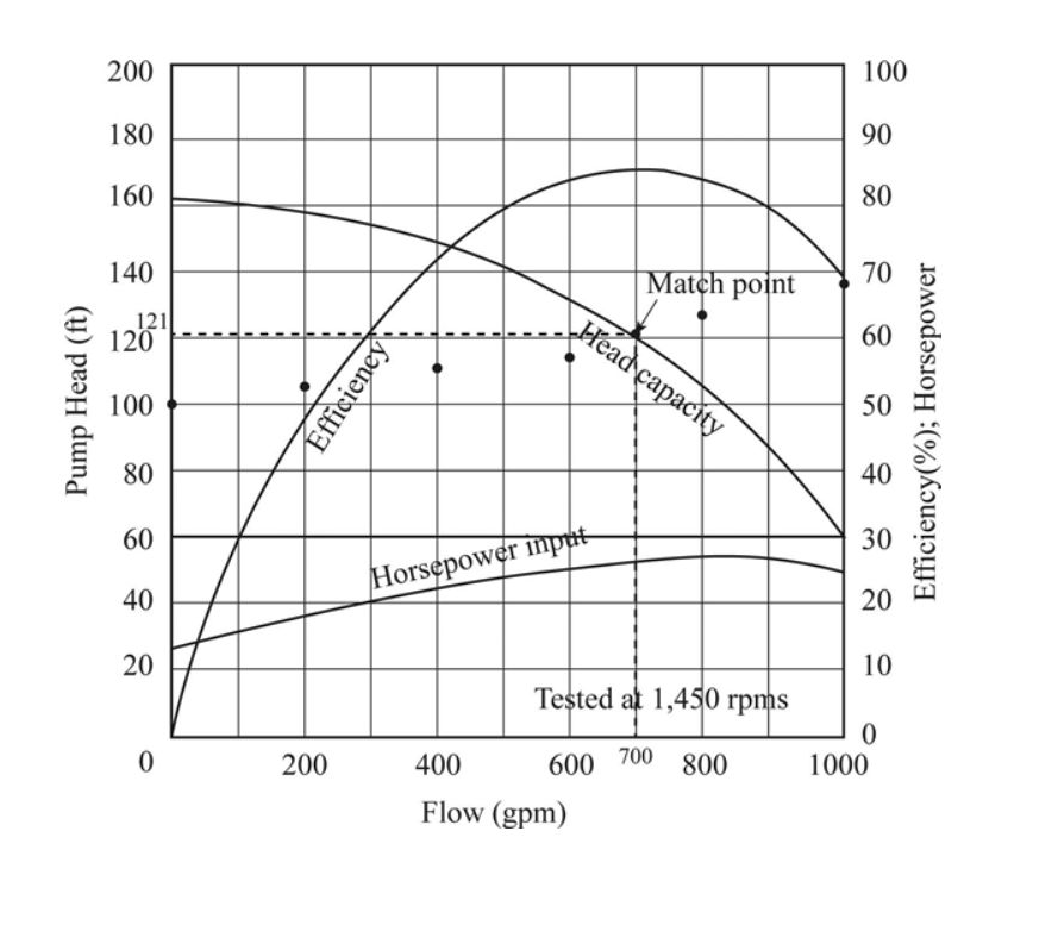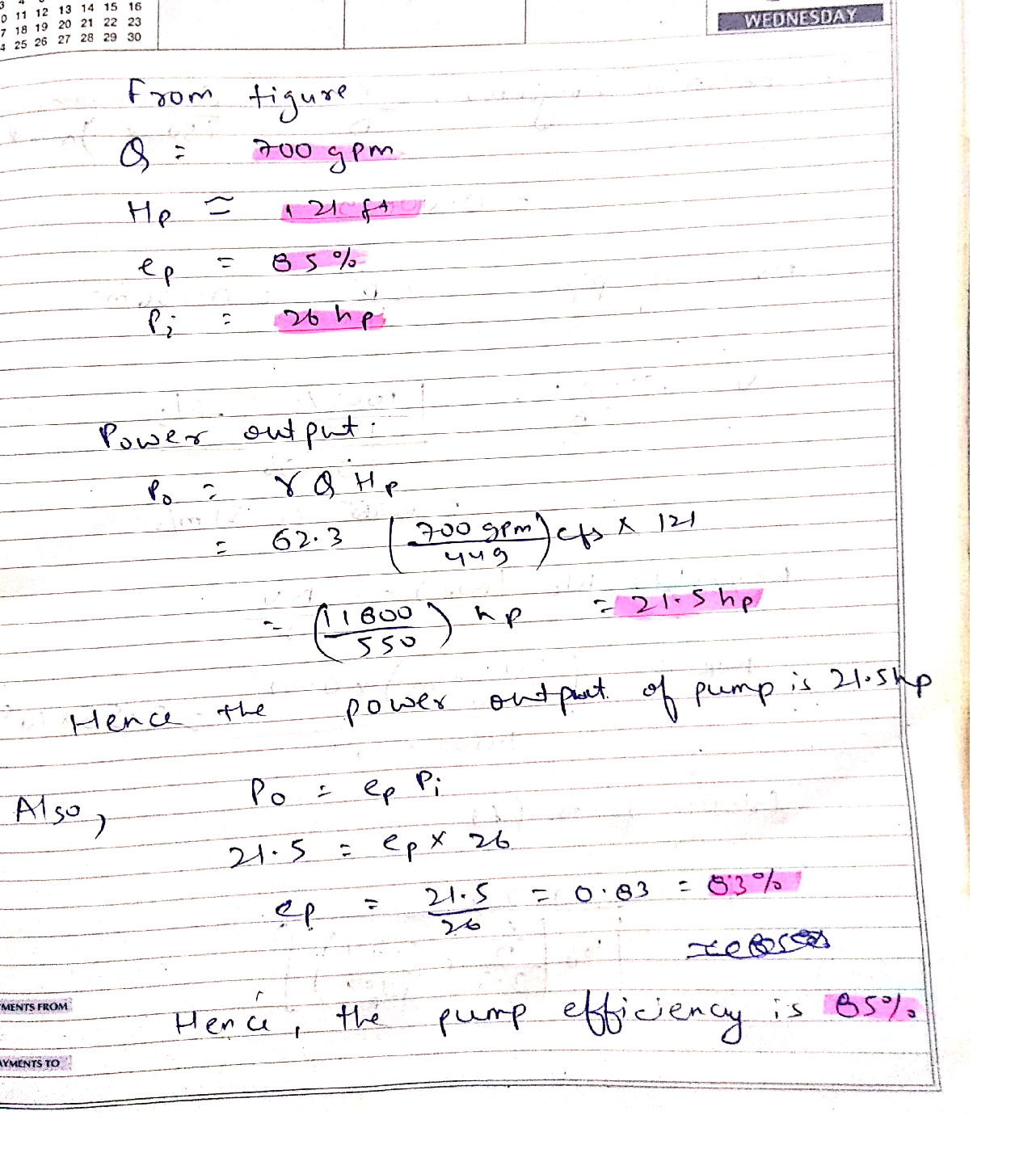Question

Hydraulics

5.11.4. A pump delivers water (68°F) from reservoir A to reservoir B, a water surface rise of 100 ft. The old 1.0-ft-diameter pipe connecting the two reservoirs has a length of 7800 ft and a Hazen Williams coefficient of 90. The pump characteristics are available in Figure P5. 11.4. Perform the following tasks.

(1) Compute the system head for Q=0 to 1000 gpm with an interval of 200 gpm by completing the table. Show your sample calculations for one Q value at least.  (2) Plot Q vs. HSH by overlaying them on Fig. P5. 11.4 and clearly mark the match point. Directly use Figure P5. 11.4 attached below.

Use the graph completed in (2) for the following tasks (3)to (6). Clearly mark your values in the graph directly and interpolate the values if needed.

(3) Determine the flow rate.  (4) Determine the pump head.  (5) Determine the brake horse power.  (6) Determine the pump efficiency. 

(7) Compute the pump output power.  (8) Verify that the pump efficiency obtained in (6) corresponds with the pump efficiency obtained from the efficiency equation.Verified### Question 45163Hydraulics

4. A closed cylindrical tank filled with water. The liquid in the top part of the piping system has a specific gravity of 0.8, and the remaining parts of the system are filled with water.
If the pressure gage reading at pointA is 60 kPa, determine the pressure inpoint B in kPa.

### Question 45162Hydraulics

3. Bourdon gages are used to measure pressure.When such a gage is attached to a closed water tank as shown below the gage reads 5 psi. What is the absolute air pressure in the tank? Assume standard atmospheric pressure of 14.7 psi.

### Question 45161Hydraulics

2. For the tank shown below, determine the reading of bottom pressure gage in psi if the top of the tank is sealed. The top gage reads -10.8 psi, and the depth of the oil is 6.25 ft.

### Question 44277Hydraulics

Consider the hydraulic lift system in the figure below, with 10 mm rod and50 mm cylinder, used to lift a 500 kg load.
A hand pump is being used with a stroke of 7 cm3 per pump actuation.How many pump cycles would be required to lift the load 100 mm high?
d) Assume you operate the hand pump at a speed of 0.3 Hz. How long would it take to lift the load 100 mm high?
e) What is the work and power required from the pump operator?
f) Assume now an electric motor is being used that pump at a rate of 3litres / min. How long would it take to lift the load 100 mm?
What is the work and power required from the electric motor?

### Question 44276Hydraulics

Explain the advantages associated with servo control using an example.

### Question 44274Hydraulics

a) Explain briefly the different valve configurations shown. (3 marks)
b) Name three typical applications in which Tandem centre spoolconfiguration is used. (1.5 marks)
What is the chief disadvantage associated with this configuration? (1.5marks)

### Question 44273Hydraulics

b) What is valve overlap? (2 marks)
c) Why is some amount of valve overlap desirable? (1 mark) overlap. (2marks)

### Question 44272Hydraulics

In a direct acting pressure relief valve, 64 bar pressure is acting on the conical dart seating area and the dart is kept closed by a spring force of 50 kg opposing the pressure.
a) Determine the bore diameter on the seat.
What will the spring force be if a pressure of 3200 kPa is acting on diameter of 10 mm seating area?

### Question 44271Hydraulics

a) Give a graphic representation of the 6 different types of pressure control valves. (4 marks)
b) The circuits below are an illustration of the working of a pressure reducing valve and a high-low system employing an unloading valve.Explain the operation of the valves. (8 marks)

### Question 44270Hydraulics

A pump delivers 40 litres/min to a 90 mm bore/30 mm rod cylinder to lift a load of 200 kg.
a) What is the pressure exerted on the piston?
b) What is the speed at which the load will be lifted?
How much power is required to lift the load at this speed?

### Submit query